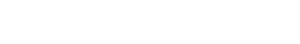# Beginning Scientific Computing

## Video Lectures and Supplementary Tutorials

This webpage is designed to provide a easy access point for all video materials related to AMATH 301: Beginning Scientific Computing (Department of Applied Mathematics, University of Washington). Each topic is broken down into weekly lectures (3 lectures per week) that are arranged topically. Included are supplementary materials related to each lecture.

### Topics Covered

Below are the mathematical subjects covered over the course of a 10-week course.

• Introduction to MATLAB and programming
• Linear Systems of Equations: Ax=b
• Singular Value Decomposition & Principal Components
• Differential and Integrable Calculus
• Differential Equations
• Fourier Transform